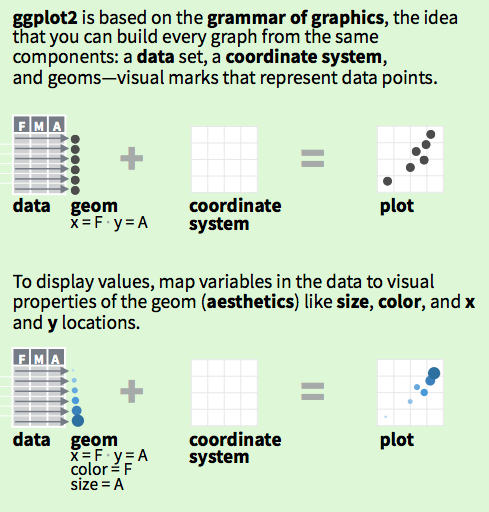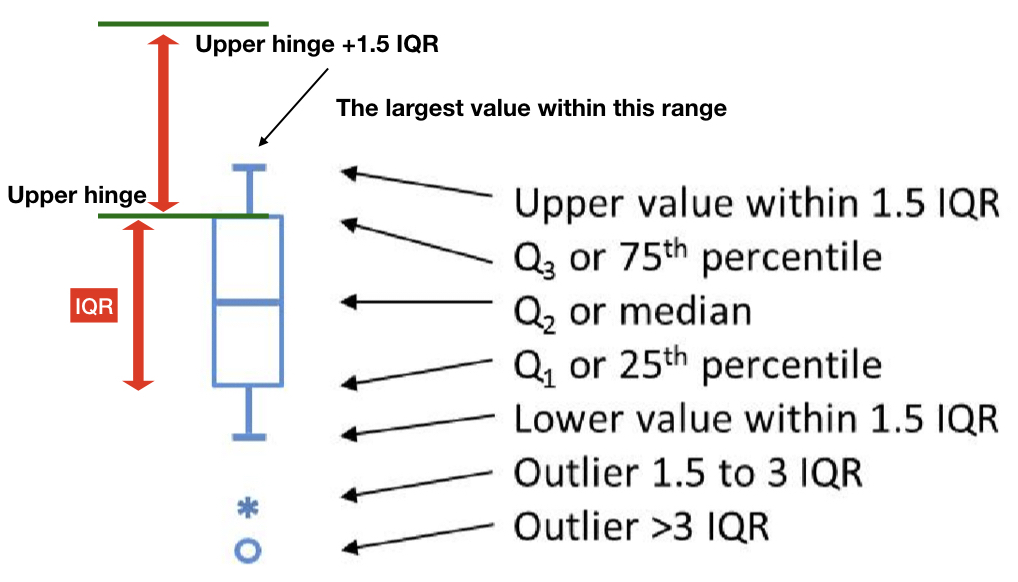# 第 2 章 ggplot2: geom

gg: grammar of graphics

## 2.1 Grammar of Graphics### 引入資料

library(readr)
subsetDataTWbank <- read_csv("https://www.dropbox.com/s/t8ulj3d7cgl8jh5/subsetDataTWbank.csv?dl=1")

### 基本語法

• 資料data: subsetDataTWbank

• 座標對應coordinate: x=西元年月,y=定存利率-一個月-固定

• 幾何圖示類型geom: line

<變數名稱>

ggplot(<資料>)+ # 設置畫布
geom_<幾何圖示類型>( # 畫上幾何圖示
aes(<座標對應>) #座標對應
)

library(ggplot2)
ggplot(subsetDataTWbank)+
geom_line(
aes(x=西元年月,y=定存利率-一個月-固定)
)

library(ggplot2)
ggplot(subsetDataTWbank)+
geom_point(
aes(x=西元年月,y=定存利率-一個月-固定)
)

• 座標對應：x=定存類型, y=利率

• 幾何圖示類型：boxplot

### 層疊概念

geom_...可以不斷以+層疊上去。

library(ggplot2)
ggplot(subsetDataTWbank)+
geom_line(
aes(x=西元年月,y=定存利率-一個月-固定)
)+
geom_point(
aes(x=西元年月,y=定存利率-一個月-固定)
)

geom裡相同的aes mapping可以移至ggplot()裡：

library(ggplot2)
ggplot(subsetDataTWbank,
aes(x=西元年月,y=定存利率-一個月-固定))+
geom_line()+
geom_point()

### 美學對應

aes＝aesthetic (美學) 在gg裡它指得是資料設計呈現的對應方式。

• color

• size

subsetDataTWbank_gather %>%
ggplot()+
geom_line(
aes(x=西元年月,y=利率,color=定存類型)
)

## 2.2 字串變數

### 類別資料

subsetDataTWbank_gather\$定存類型 %>%
as.factor %>% levels

subsetDataTWbank_gather %>%
mutate(
定存類型=factor(定存類型,levels=c("定存利率-一個月-固定","定存利率-二年期-固定","定存利率-三年期-固定"))
) -> subsetDataTWbank_gather

subsetDataTWbank_gather %>%
ggplot()+
geom_boxplot(aes(x=定存類型,y=利率))

• 會以factor處理。

• 幾何位置會以其levels順序決定。

• levels順序可以factor(..., levels=類別文字向量)中的類別文字向量元素值序改變。

### 時間資料

1. 非西元日期字串改成西元日期字串。

2. 西元日期字串使用lubridate套件改成date time class。

twCalendar <- c("民國88年11月1日","民國88年12月1日","民國89年1月1日")

twCalendar %>%
# 取出字串中的數字部份
stringr::str_extract_all("[:digit:]+") %>%
# 將每個日期的「年」數字加上1991再合併年月日成為yyyy-mm-dd格式
purrr::map_chr(
function(x) {
x1=as.integer(x[])+1911
x[]=as.character(x1)
stringr::str_c(x,collapse="-")
}
) -> westernCalendar

westernCalendar %>%
lubridate::ymd() -> datetimeCalendar
class(datetimeCalendar)

subsetDataTW範例

subsetDataTWbank %>%
mutate(
年月=年月 %>%
stringr::str_extract_all("[:digit:]+") %>%
purrr::map_chr(
function(x) {
x1=as.integer(x[])+1911
x[]=as.character(x1)
stringr::str_c(x,collapse="-")
}
),
年月日=stringr::str_c(年月,"01",sep="-"),
西元年月日=lubridate::ymd(年月日)
)

## 2.3 Geom介紹：boxplot## 2.4 設定中文字體

Package: showtext

### 查查有什麼字體

library(showtext)
font_files()

### 設定字體

font_add("QYuan","cwTeXQYuan-Medium.ttf") # 新增字體
showtext_auto(enable=TRUE) #啟用字體
subsetDataTWbank %>% ggplot(aes(x=西元年月,y=定存利率-一個月-固定))+
geom_line()+
theme(text=element_text(size=10,  family="QYuan"))+
labs(x="西元年月",y="利率：%")

## 2.5 圖形存檔

subsetDataTWbank %>% ggplot(aes(x=西元年月,y=定存利率-一個月-固定))+
geom_line()+
theme(text=element_text(size=10,  family="QYuan")) -> figureOut
ggsave(filename="Interest Rate.png",figureOut)`